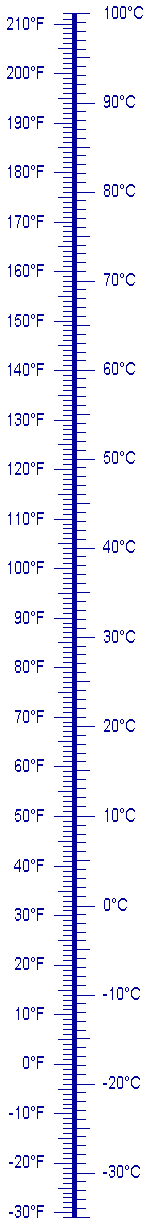Home >> Index >> Metric Conversions

# Metric Conversions

 Main Index Currency Special Characters GMT Time Visitors' Comments Maps About • Home • Search What's New?

To convert:

 kilometers to miles multiply by 0.6215 miles to kilometers multiply by 1.609

 meters to inches multiply by 39.37 inches to meters multiply by 0.0254 meters to feet multiply by 3.281 feet to meters multiply by 0.3048 centimeters to inches multiply by 0.3937 inches to centimeters multiply by 2.54 millimeters to inches multiply by 0.03937 inches to millimeters multiply by 25.4

 square kilometers to square miles multiply by 0.3861 square miles to square kilometers multiply by 2.59 hectares to acres multiply by 2.47 acres to hectares multiply by 0.405 square miles to acres multiply by 640 acres to square miles multiply by 0.0015625 square meters to square yards multiply by 1.196 square yards to square meters multiply by 0.8361 square meters to square feet multiply by 10.76 square feet to square meters multiply by 0.0929 square centimeters to square inches multiply by 0.155 square inches to square centimeters multiply by 6.45

 cubic meters to cubic yards multiply by 1.307 cubic yards to cubic meters multiply by 0.7651 cubic centimeters to cubic inches multiply by 0.06102 cubic inches to cubic centimeters multiply by 16.387

 liters to quarts multiply by 1.057 quarts to liters multiply by 0.9461 liters to gallons multiply by 0.2642 gallons to liters multiply by 3.785 liters to fluid ounces multiply by 33.81 fluid ounces to liters multiply by 0.02957

 metric tons to U.S. tons multiply by 1.102 U.S. tons to metric tons multiply by 0.9074 kilograms to pounds multiply by 2.2046 pounds to kilograms multiply by 0.4536 grams to ounces multiply by 0.035 ounces to grams multiply by 28.6 milligrams to grains multiply by 0.015 grains to milligrams multiply by 67 newtons to pounds multiply by 0.2248 pounds to newtons multiply by 4.448

 inches of mercury to kilopascals multiply by 3.386 kilopascals to inches of mercury multiply by 0.2953

 If you were looking for something here and didn't find it, let me know and I will add it for next time. tom@tomzap.com

Temperature| Top of page | Main index | What's new |
The Pacific Coast of Mexicowww.tomzap.comTom Penick:  tom@tomzap.com